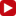Technologically Embodied
Geometric Functions
Complex Multiplication Part 1: Dilate a Complex Number

In this investigation you will explore the effect of dilation of complex numbers about the origin, and describe your results numerically.

You will start by dilating real numbers using several different scale factors, follow up by dilating imaginary numbers, and conclude by dilating complex numbers.

As a result of your investigation, you will describe the numeric effect of dilation on both the rectangular (x + yi) and the polar (r, θ) forms of expressing the complex number.

• Page 1: What happens when you dilate a number on the real (x) axis by a scale factor like 3, 0.5, or -2? Describe the result numerically.
• Page 2: What happens when you dilate a number on the imaginary (y) axis by a scale factor? Describe the result numerically.
• Page 3: What happens when you dilate an arbitrary complex number (anywhere on the complex plane) by a scale factor?
• Conclusion: Describe the numeric effect of dilation on both the rectangular (x + yi) and the polar (r, θ) forms of expressing the complex number being dilated.

•
XPage 1Page 2Page 3

Release Information and Rights

Update History: